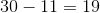# ISEE Upper Level Math : How to use a Venn Diagram

## Example Questions

### Example Question #1 : Data Analysis And Probability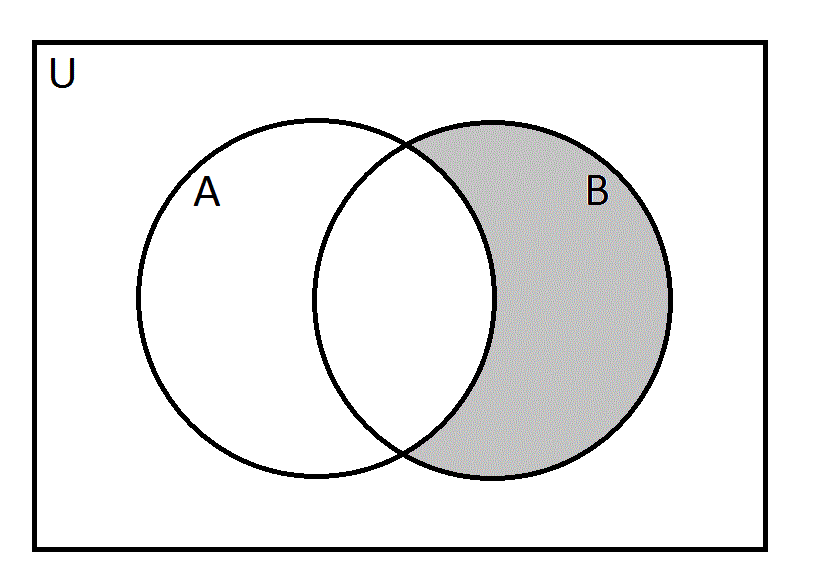Refer to the above Venn diagram.

Define universal set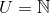, the set of natural numbers.

Define setsandas follows: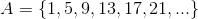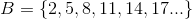Which of the following numbers is an element of the set represented by the gray area in the diagram?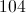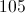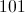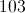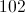Explanation:

The gray area represents the set of all elements that are inbut not in.is the set of integers that, when divided by 3, yield remainder 2. Therefore, we can eliminate 102 and 105, both multiples of 3, and 103, which, when divided by 3, yields remainder 1.is the set of integers that, when divided by 4, yield remainder 1. Since we do not want an element from this set, we can eliminate 101, but not 104.

104 is the correct choice.

### Example Question #2 : Data Analysis And Probability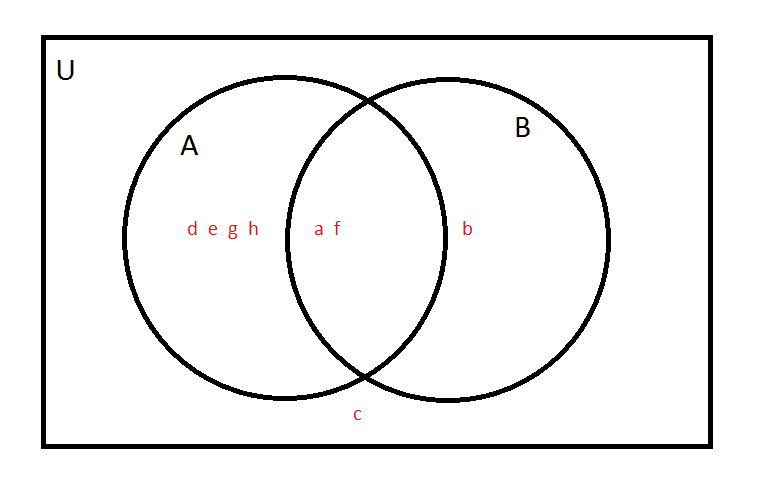In the above Venn diagram, the universal set is defined as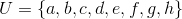. Each of the eight letters is placed in its correct region.

What is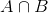?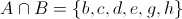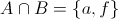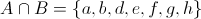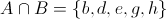Explanation:is the intersection of setsand- that is, the set of all elements of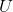that are elements of bothand. We want all of the letters that fall in both circles, which from the diagram can be seen to beand. Therefore,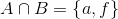### Example Question #3 : Data Analysis And ProbabilityIn the above Venn diagram, the universal set is defined as. Each of the eight letters is placed in its correct region. Which of the following is equal to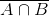?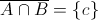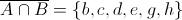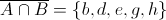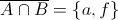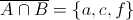Explanation:is the complement of- the set of all elements innot inis the intersection of setsand- that is, the set of all elements ofthat are elements of bothand. Therefore,is the set of all elements that are not in bothand, which can be seen from the diagram to be all elements exceptand. Therefore,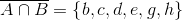.

### Example Question #4 : Data Analysis And ProbabilityIn the above Venn diagram, the universal set is defined as. Each of the eight letters is placed in its correct region. Which of the following is equal to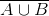?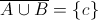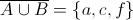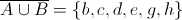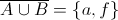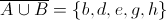Explanation:is the complement of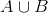- the set of all elements innot in.is the union of setsand- the set of all elements in eitheror. Therefore,is the set of all elements in neithernor, which can be seen from the diagram to be only one element -. Therefore,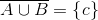### Example Question #5 : Data Analysis And Probability

The following Venn diagram depicts the number of students who play hockey, football, and baseball. How many students play football and baseball?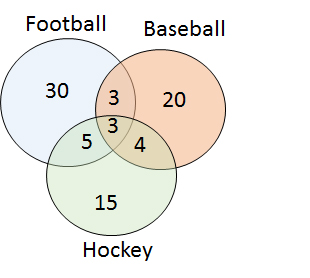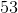Explanation:

The number of students who play football or baseball can by finding the summer of the number of students who play football alone, baseball alone, baseball and football, and all three sports.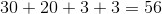### Example Question #6 : Data Analysis And Probability

A class ofstudents was asked whether they have cats, dogs, or both.The results are depicted in the following Venn diagram. How many students do not have a dog?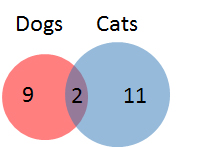Explanation:

First, calculate the number of students with a dog: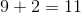Next, subtract the number of students with a dog from the total number of students.